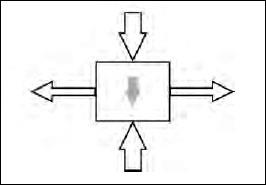NextPrevious

# What is water pressure?

Pressure is the force divided by the area over which it is exerted. In 1647 the French scientist Blaise Pascal (1623–1662) recognized that water exerts the same pressure in all directions. This statement is known as Pascal’s Principle.

To understand Pascal’s Principle, think of a small cube of water as shown on page 96. The grey arrow in the middle is the force of gravity on the cube. As a result the total downward force of the cube is larger than the upward force.

Now, by Newton’s Third Law, the outward force of the water is equal to the inward force on the water. What exerts this force? If the cube is at the edge of the container, then the container exerts the force. You can check that for yourself. Use an empty fluid container—say a juice box or milk carton. Punch a hole in one side. Pour water into the container. To keep the water from leaving through the hole you have to exert a force with your thumb. The same would be true if the hole were in the bottom. What exerts the downward force on the top of the cube? If the cube is not at the top surface, then the force is exerted by water above it. If it is on the top, then air pressure, which we will discuss shortly, exerts this force.

Because the downward force exerted by the fluid is greater than the upward force, the force, and hence the pressure, increases as the depth of the fluid increases. So the pressure at the bottom of a container of fluid is greater than at the top. The amount of increase in pressure is given by the product of the density, ρ, the gravitation field strength, g, and the depth, h, or ρgh. That is, the pressure on the bottom, Pbottom = Ptop ρgh.Close

This is a web preview of the "The Handy Physics Answer Book" app. Many features only work on your mobile device. If you like what you see, we hope you will consider buying. Get the App